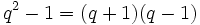# General linear group of degree two

## Definition

### For a unital ring

The general linear group of degree two over a unital ring$R$ is defined as the group, under matrix multiplication, of invertible$2 \times 2$ matrices with entries in$R$. It is denoted$GL(2,R)$.

### For a commutative unital ring

When$R$ is a commutative unital ring, a$2 \times 2$ matrix over$R$ being invertible is equivalent to its determinant being an invertible element of$R$, so the general linear group$GL(2,R)$ is defined as the following group of matrices under matrix multiplication:$GL(2,R) := \left \{ \begin{pmatrix} a & b \\ c & d \\\end{pmatrix} \mid a,b,c,d \in R, ad - bc \mbox{ is an invertible element of } R \right \}$

### For a field

For a field$K$, an element is invertible iff it is nonzero, so the general linear group$GL(2,K)$ is defined as the following group of matrices under matrix multiplication:$GL(2,K) := \left \{ \begin{pmatrix} a & b \\ c & d \\\end{pmatrix} \mid a,b,c,d \in K, ad - bc \ne 0 \right \}$

### For a prime power

For a prime power$q$,$GL(2,q)$ or$GL_2(q)$ denotes the general linear group of degree two over the finite field (unique up to isomorphism) with$q$ elements. This is a field of characteristic$p$, where$p$ is the prime number whose power is$q$.

## Particular cases

### Finite fields

Common name for general linear group of degree two Field Size of field Order of group
symmetric group:S3 field:F2 2 6
general linear group:GL(2,3) field:F3 3 48
direct product of A5 and Z3 field:F4 4 180
general linear group:GL(2,5) field:F5 5 480

### Infinite rings and fields

Name of ring/field Common name for general linear group of degree two
Ring of integers$\mathbb{Z}$ general linear group:GL(2,Z)
Field of rational numbers$\mathbb{Q}$ general linear group:GL(2,Q)
Field of real numbers$\mathbb{R}$ general linear group:GL(2,R)
Field of complex numbers$\mathbb{C}$ general linear group:GL(2,C)

## Arithmetic functions

Here,$q$ denotes the order of the finite field and the group we work with is$GL(2,q)$.$p$ is the characteristic of the field, i.e., it is the prime whose power$q$ is.

Function Value Explanation
order$\! (q^2 - 1)(q^2 - q) = q^4 - q^3 - q^2 + q = q(q + 1)(q-1)^2$$q^2 - 1$ options for first row,$q^2 - q$ options for second row.
See order formulas for linear groups of degree two
exponent$\! p(q^2 - 1) = p(q-1)(q+1)$ There is an element of order$q^2 - 1$ and an element of order$p(q - 1)$. All elements have order dividing$p(q - 1)$ or$q^2 - 1$.
number of conjugacy classes$\! q^2 - 1 = (q + 1)(q-1)$ There are$q(q-1)$ conjugacy classes of semisimple matrices and$q - 1$ conjugacy classes of matrices with repeated eigenvalues.

## Group properties

Property Satisfied? Explanation
abelian group No The matrices$\begin{pmatrix} 1 & 1 \\ 0 & 1 \\\end{pmatrix}$ and$\begin{pmatrix} 0 & 1 \\ 1 & 0 \\\end{pmatrix}$ don't commute.
nilpotent group No$PSL(2,q)$ is simple for$q \ge 4$, and we can check the cases$q = 2, 3$ separately.
solvable group Yes if$q = 2,3$, no otherwise.$PSL(2,q)$ is simple for$q \ge 4$.
supersolvable group Yes if$q = 2$, no otherwise.$PSL(2,q)$ is simple for$q \ge 4$, and we can check the cases$q = 2, 3$ separately.

## Elements

### Conjugacy class structure (case of a field)

Nature of conjugacy class Eigenvalues Characteristic polynomial Minimal polynomial What set can each conjugacy class be identified with? (rough measure of size of conjugacy class) What can the set of conjugacy classes be identified with (rough measure of number of conjugacy classes) What can the union of conjugacy classes be identified with? Semisimple? Diagonalizable over$K$?
Diagonalizable over$K$ with equal diagonal entries, hence a scalar$\{a,a \}$ where$a \in K^\ast$$(x - a)^2$ where$a \in K^\ast$$x - a$ where$a \in K^\ast$ one-point set$K^\ast$$K^\ast$ Yes Yes
Diagonalizable over$K$ with distinct diagonal entries$\lambda, \mu$ (interchangeable) distinct elements of$K^\ast$$x^2 - (\lambda + \mu)x + \lambda \mu$ Same as characteristic polynomial set of decompositions of a fixed two-dimensional vector space over$K$ as a direct sum of one-dimensional subspaces the set$\binom{K^\ast}{2}$  ? Yes Yes
Diagonalizable over a quadratic extension of$K$ but not over$K$ itself. Must necessarily have no repeated eigenvalues. Pair of conjugate elements of some separable quadratic extension of$K$$x^2 - ax + b$, irreducible Same as characteristic polynomial  ?  ?  ? Yes No
Not diagonal, has Jordan block of size two with eigenvalue in$K$$a$ (multiplicity two) where$a \in K^\ast$$(x - a)^2$ where$a \in K^\ast$ Same as characteristic polynomial  ?  ?  ? No No
Not diagonal, has Jordan block of size two with eigenvalue not in$K$$a$ (multiplicity two) where$a$ is in a purely inseparable quadratic extension of$K$, so$a^2 \in K$. This case arises only when$K$ has characteristic two and is not a perfect field$x^2 - t$,$t$ a non-square in$K^\ast$,$K$ has characteristic two Same as characteristic polynomial  ? ?  ? No No

## Subgroup-defining functions

Subgroup-defining function Value Explanation
Center The subgroup of scalar matrices. Cyclic of order$q - 1$ Center of general linear group is group of scalar matrices over center.
Commutator subgroup Except the case of$GL(2,2)$, it is the special linear group of degree two, which has index$q - 1$. Commutator subgroup of general linear group is special linear group

## Quotient-defining functions

Subgroup-defining function Value Explanation
Inner automorphism group Projective general linear group of degree two Quotient by the center, which is the group of scalar matrices.
Abelianization This is isomorphic to the multiplicative group of the field. Quotient by the commutator subgroup, which is the special linear group, which is the kernel of the determinant map that surjects to the multiplicative group of the field.